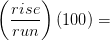# 1.3: Tips for Measuring Slope on Contour Maps

$$\newcommand{\vecs}{\overset { \rightharpoonup} {\mathbf{#1}} }$$ $$\newcommand{\vecd}{\overset{-\!-\!\rightharpoonup}{\vphantom{a}\smash {#1}}}$$$$\newcommand{\id}{\mathrm{id}}$$ $$\newcommand{\Span}{\mathrm{span}}$$ $$\newcommand{\kernel}{\mathrm{null}\,}$$ $$\newcommand{\range}{\mathrm{range}\,}$$ $$\newcommand{\RealPart}{\mathrm{Re}}$$ $$\newcommand{\ImaginaryPart}{\mathrm{Im}}$$ $$\newcommand{\Argument}{\mathrm{Arg}}$$ $$\newcommand{\norm}{\| #1 \|}$$ $$\newcommand{\inner}{\langle #1, #2 \rangle}$$ $$\newcommand{\Span}{\mathrm{span}}$$ $$\newcommand{\id}{\mathrm{id}}$$ $$\newcommand{\Span}{\mathrm{span}}$$ $$\newcommand{\kernel}{\mathrm{null}\,}$$ $$\newcommand{\range}{\mathrm{range}\,}$$ $$\newcommand{\RealPart}{\mathrm{Re}}$$ $$\newcommand{\ImaginaryPart}{\mathrm{Im}}$$ $$\newcommand{\Argument}{\mathrm{Arg}}$$ $$\newcommand{\norm}{\| #1 \|}$$ $$\newcommand{\inner}{\langle #1, #2 \rangle}$$ $$\newcommand{\Span}{\mathrm{span}}$$$$\newcommand{\AA}{\unicode[.8,0]{x212B}}$$

To calculate %slope of the land from contour maps, we still need to determine the rise and run. On a map, the rise is the difference in elevation between two points. The run is the horizontal distance between two points. Map distance is always horizontal distance.

The Rise is the difference in elevation between two points. Using the elevations printed on the map and the contour line interval, an elevation can be determined for the top and bottom of the slope in question. It generally works better to simply determine the elevation at each point and subtract rather than to “count the contour lines” between the two points. Doing the latter often results in rounding errors or double counting a contour line that can throw slope readings off by 10% or more.

To determine the run, the map distance is measured between the two points, and converted to the same units as the elevation. If difference in elevation is measured in feet, distance should also be calculated in feet. If difference in elevation is measured in meters, distance should also be calculated in meters.

Example $$\PageIndex{1}$$:

An excerpt from a contour map is shown below. To determine the %slope from Point A to Point B, we must first determine the rise and run.Rise:

If the map has 40’ contour intervals, then Point A is located at 3000 feet. Point B is located at 2840 feet. Therefore:

Rise = change in elevation = top (Point A elevation) minus bottom (Point B elevation): 3000’ – 2840’ = 160 feet

Run:

If the map scale is 1inch = 500 feet, then the run is calculated as follows:

The map distanced measured 1.8 inches with an engineer’s scale.900 feet

%slope:%slope18%

From Point A to Point B, the slope is –18% (downhill); from B to A the slope is +18% (uphill).

This page titled 1.3: Tips for Measuring Slope on Contour Maps is shared under a CC BY license and was authored, remixed, and/or curated by Joan DeYoung (OpenOregon) .